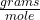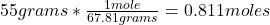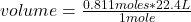## At STP, what is the volume of 55.0 g of BF3?

Question

At STP, what is the volume of 55.0 g of BF3?

in progress 0
2 months 2021-07-23T16:41:27+00:00 1 Answers 5 views 0

The volume of 55 grams of BF₃ is 18.1664 L.

Explanation:

If the molar mass of compound BF₃ is 67.81, then the number of moles 55 grams of compound contains is calculated as:The STP conditions refer to the standard temperature and pressure. Pressure values at 1 atmosphere and temperature at 0 ° C are used and are reference values for gases. And in these conditions 1 mole of any gas occupies an approximate volume of 22.4 liters.

Then it is possible to apply the following rule of three: if by definition of STP 1 mole of a compound occupies 22.4 L, 0.811 moles of the compound occupies how much volume?volume= 18.1664 L

The volume of 55 grams of BF₃ is 18.1664 L.